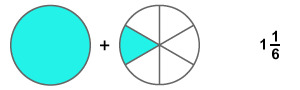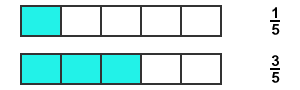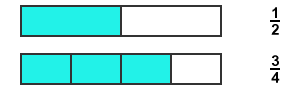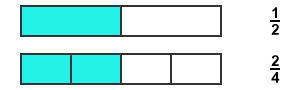Types of fractions

Fractions are mainly classified into three types by the system of their formation.

1.

Proper fractionA fraction which contains less value in numerator than denominator is called proper fraction.

2.

Improper fractionA fraction which contains greater value in numerator than denominator is called improper fraction.

3.

Mixed fractionA fraction which contains a whole and a fraction is called mixed fraction.

Fractions are also classified into three types by comparing them.

1.

Like fractionsThe fractions which have same denominators are called like fractions.

2.

Unlike fractionsThe fractions which have different denominators are called unlike fractions.

3.

Equivalent fractionsThe fractions which have equal numerical value are called equal fractions.

Latest Math Topics
Latest Math Problems
Email subscription
Math Doubts is a best place to learn mathematics and from basics to advanced scientific level for students, teachers and researchers. Know more